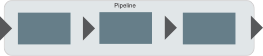XSL-FO - WordML - XSLT

# Pipelines

Pipelines are the primary basic concept of XProc. A pipeline always has an input and an output from which the output document is produced. The pipeline consists of individual steps which are to be considered as partial processing steps.Figure: XProc pipeline

A pipeline may become very long and complex. There are two possibilities to generate them. You can either use <p:declare-step> or <p:pipeline>.

## p:declare-step

With the help of <p:declare-step>, pipelines (or steps) are generated.

``````<p:declare-step
name? = NCName
type? = QName
psvi-required? = boolean
xpath-version? = string
exclude-inline-prefixes? = prefix list
version? = string>
(p:input |
p:output |
p:option |
p:log |
p:serialization)*,
((p:declare-step |
p:pipeline |
p:import)*,
subpipeline)?
</p:declare-step>``````

As you can see in the code example, a <p:declare-step> can be provided with various settings. Later the <p:declare-step> element will be discussed again and the individual attributes will be described in more detail. At first it is relevant that a name can be assigned to the entire pipeline by the “name“ attribute (this aspect is important for the linking of steps).

In the “version“ attribute the current version of XProc is entered. This is relevant for the processor being used (e.g. Calumet).

In XProc a pipeline is treated like a step since it can also appear for example in another pipeline as a step.

### Example

In the following example a trivial pipeline is generated with the help of <p:declare-step>.

``````<?xml version="1.0" encoding="UTF-8"?>
<p:declare-step xmlns:p="http://www.w3.org/ns/xproc" xmlns:c="http://www.w3.org/ns/xproc-step" version="1.0">
<p:input port="source" sequence="true"/>
<p:output port="result" sequence="true"/>
<p:identity/>
</p:declare-step>``````

Firstly, the two XProc namespaces are generated with its prefixes “p:“ and “c:“. In the “version“ attribute the value “1.0“ is indicated. So, XProc with the version number 1.0 is used. Finally the name “TestPipeline“ is assigned to the pipeline. When indicating an input and an output port, the “sequence=true“ attribute shall be used.

If sequence is not “true“, an error would occur in the pipeline shown in the figure. A sequence means that several documents (or no documents) can be loaded at the respective input or output port. If the value is “false“, exactly one document is expected. In the example nothing is loaded, which means that an error would occur when using “sequence=false“. Per default the value is “false“, so it has to be explicitily switched to “true“.

Then, a <p:identity> step is executed. So a pipeline has been successfully described. As result only the content of the input port (which means nothing in this example) is outputted. Here, almost all of the pipelines are defined exclusively with <p:declare-step>.

## p:pipeline

With <p:pipeline>, pipelines can be generated in XProc.

``````<p:pipeline
name? = NCName
type? = QName
psvi-required? = boolean
xpath-version? = string
exclude-inline-prefixes? = prefix list
version? = string>
(p:input |
p:output |
p:option |
p:log |
p:serialization)*,
(p:declare-step |
p:pipeline |
p:import)*,
subpipeline
</p:pipeline>``````

At first sight, <p:pipeline> seems identical in construction to <p:declare-step> because it has the same attributes and information. The difference to <p:declare-step> is that <p:pipeline> is automatically equipped with a primary input port called “source“, a parameter port called “parameter“ and a primary output port called “result“. So, these do not have to be specified manually. Since these ports are required by most steps, it is a facilitation (because you do not have to write so much).

### Example

In the following example the same trivial pipeline from the previous example (see <p:declare-step>) is generated with the help of <p:pipeline>.

``````<?xml version="1.0" encoding="UTF-8"?>
<p:pipeline xmlns:p="http://www.w3.org/ns/xproc" xmlns:c="http://www.w3.org/ns/xproc-step" version="1.0" name="TrivialPipeline">
<p:identity/>
</p:pipeline>``````

Since <p:pipeline> does not require the definition of the standard ports, the indication of the wanted step (<p:identity>) is sufficient. However, this variant requires an input (e.g. a document) because the predefined ports of <p:pipeline> have the sequence value “false“. Otherwise some processors would create errors (when using “sequence=false“, exactly one document is expected).